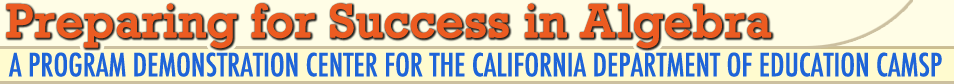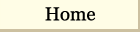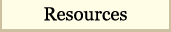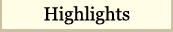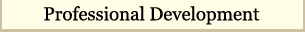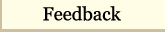CONTENT: Pedagogy >> TOPIC: Questioning Strategies >> LEVEL: 5, 6, 7, 8, Algebra >> TITLE: Distance = Rate * Time Next >>

This section correlates with the Pedagogy Powerpoint.

## Pedagogy

The pedagogy section is designed to pick up on the concepts in the math section, mainly distance, rate, and time. At the fifth grade level, it's given two of the quantities what happens at the third one. At the sixth grade level, it's comparing two different objects traveling. At the seventh/Algebra level it's looking at rate of change and slope.

Essential Questions
• How does types of teacher questioning impact student understanding of the content?
• How does the use of visual models help conceptual understanding?
• How does the use of technology in the classroom enhance students understanding of mathematics?

Next >>